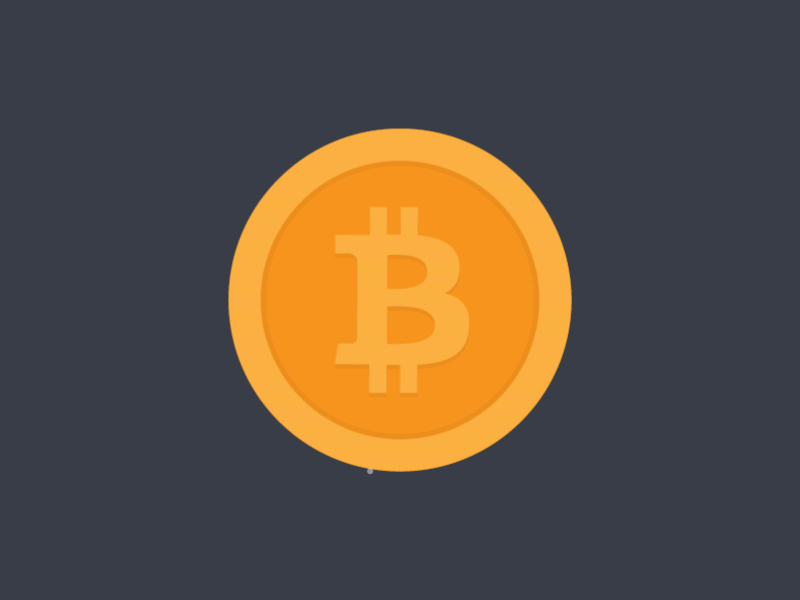# Random Starscapes

In the world of programing, there is alot of order and keeping things in place.
But a lot of times it is needed to be a little random. for example:flipping coins.
Since the advent of fractal worlds in video games, random number genorators have been nessesary.
One cool thing that I have found useful for randomness is making a star field.
Here is my example:
(click on the link again to randomize)

here is my code:

var ctx = myCanvas.getContext("2d");
function RandInt(up_to) {
n = Math.floor(Math.random() * up_to);
return(n);
}
function CircleRandColour (x, y, radius) {
r = RandInt(5); // r will be 0, 1, 2, 3, or 4
if (r == 0) {
ctx.fillStyle = "white";
}
else if (r == 1) {
ctx.fillStyle= "gray";
}
else if (r == 2) {
ctx.fillStyle= "yellow";
}
else if (r == 3) {
ctx.fillStyle= "blue";
}
else if (r == 4) {
ctx.fillStyle= "orange";
}
ctx.beginPath();
ctx.arc(x, y, 1, 0, Math.PI * 2);
ctx.fill();
}
for (var i=0; i<=1000; i++) {
CircleRandColour (RandInt(myCanvas.width), RandInt(myCanvas.height), RandInt(30)+1);
}